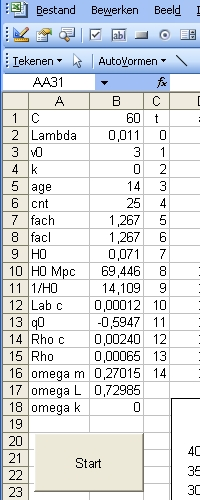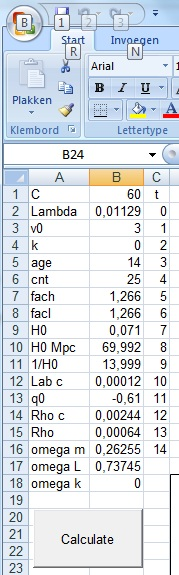# Operation Excel program friedmann's equation.xls & Copy

## Introduction

The purpose of this document is to explain how to operate and how to modify the program friedmann's equation.xls
For a copy of the Excel program select:friedmann's equation.xls.zip
Modification of the program depents on the Excel program used:
There are two major possibilities:
Excel 2003 or Excel 2007
The following two pictures allows you to select which type of Excel program is installed:Excel 2003Excel 2007

## Operation

1. The program friedmann's equation.xls contains 3 sheets or pages with the name:
Lambda < 0 , Lamba = 0 and Lamba > 0
The programs displayed are identical. The parameters tested are different. There is also a sheet (page) with the name Graphic, but that one is of no importance.
2. In order to start the program select the START or CALCULATE pushbutton.
Execution of the program goes more or less in two phases:
1. In the first phase the major parameters of the friedmann equation are calculated as a function of the parameters C, Lambda, k and age.
2. In the second phase the path of a light ray is calculated.
This calculation goes in 25 cycles. The parameter cnt shows how far the calculation is going.
3. Input to the program are the following parameters:
C, Lambda, v0, k, age and cnt
1. C defines the total mass in the Universe. C/R^3 is the density.
2. Lambda is the Cosmological Constant.
3. V0 is the intitial speed. dR/dt at t=0
4. k k is the curvature. Physical values are: k=-1 k=0 (flat) and k=1. Non whole numbers can also be tested.
5. age is the age of the Universe in billion of Light years. Non whole numbers can also be tested.
6. cnt is the number cycles to calculate the path of the lightray. In case cnt=0 the path shows the initial condition. Values to try are cnt = 2.
4. Output of the program are the following single parameters:
H0, H0 in Mpc, 1/H0, Lambda critical, q0, Rho critical, Rho, Omega(M), Omega(Lambda) and Omega(k)
1. H0 is the Hubble parameter at t=0
2. H0 in Mpc is the Hubble parameter at t=0 in Mpc
3. 1/H0 is the Hubble time in billion lightyears.
4. Lambda critical (Lambda c) is defined as: 3*H0^2/(2*pi). Lambda critical is a pure mathematical parameter when Lambda<>0 and can not be measured in general.
5. q0 is defined as:
5. Output of the program are the following arrays or columns:
1. t This column contains the time since the Big Bang in billion of light years.
2. a This is the first term in the friedmann equation: = C/R
3. b This is the second term in the friedmann equation: = Lambda*R^2/3
4. r This is the friedmann equation: = C/R + Lambda*R^2/3 - k

## Modification

### Modification with Excel 2003

In order to modify the program select Picture and Select either drawing or Visual Basic The result should be an icon which shows a triangle, a pencil and a ruler
Select this icon. The icon should change to orange.
Select the START or CALCULATE pushbutton.
Now you will see the program (part of)
Select Module1

### Modification with Excel 2007

In order to modify the program select the development TAB.
The banner should show an icon with: a triangle, a pencil and a ruler and the text : Design mode.
Select this icon. The icon should change to orange.
Select the START or CALCULATE pushbutton.
Now you will see the program (part of)
Select Module1